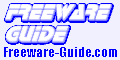Geometria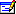Geometria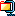2.4 MB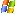XP/2003/Vista/7/8 Geometria provides a graphic interface for creating and solving problems in solid geometry. At any given time, the Geometria user is either a problem creator (a.k.a. teacher) or a problem solver (a.k.a. student). Both problems and solutions are called documents. Documents are saved in files. Users engage in a Geometria process as follows: - The creator creates a problem, provides it with an answer and saves the problem to a file. - The solver opens the problem file and starts a new solution to the problem. - The solution consists of a number of actions. The actions are recorded in the solution log. - The solver answers the problem. The solver's answer is considered correct if it matches the creator's. A correct answer concludes the solution log. The solver saves the solution to a file. - The solution can be played back by opening the file and following the solver's actions. Note that Geometria performs no semantic analysis of the problem in order to determine if the creator's answer is actually correct. The answer may be incorrect or even make no sense at all. The problem may make no sense either. The desktop version of GEOMETRIA requires Java Runtime Environment 1.5 or higher installed on your computer.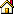Home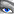Screenshot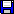Download

© The Freeware Guide